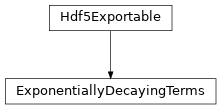# ExponentiallyDecayingTerms¶

Inheritance DiagramMethods

 ExponentiallyDecayingTerms.__init__(L) Initialize self. Add an exponentially decaying long-range coupling. Add terms from onsite_terms to an MPOGraph. Load instance from a HDF5 file. Maximum range of the couplings. Export self into a HDF5 file. Convert self into a TermList.
class tenpy.networks.terms.ExponentiallyDecayingTerms(L)[source]

Represent a sum of exponentially decaying (long-range) couplings.

MPOs can represent translation invariant, exponentially decaying long-range terms of the following form with a single extra index of the virtual bonds:

$sum_{i \neq j} lambda^{|i-j|} A_i B_j$

For 2D cylinders (or ladders), we need a slight generalization of this, where the operators act only on a subset of the sites in each unit cell, given by a 1D array subsites:

$strength sum_{i < j} lambda^{|i-j|} A_{subsites[i]} B_{subsites[j]}$

Note that we still have |i-j|, such that this will give uniformly decaying interactions, independent of the way the MPS winds through the 2D lattice, as long as subsites is sorted. An easy example would be a ladder, where we want the long-range interactions on the first rung only, subsites = lat.mps_idx_fix_u(u=0), see mps_idx_fix_u().

Parameters

L (int) – Number of sites.

L

Number of sites.

Type

int

exp_decaying_terms

Each tuple (strength, opname_i, opname_j, lambda, subsites, opname_string) represents one of the terms as described above; see add_exponentially_decaying_coupling() for more details.

Type

list of tuples

add_exponentially_decaying_coupling(strength, lambda_, op_i, op_j, subsites=None, op_string='Id')[source]

Add an exponentially decaying long-range coupling.

$strength sum_{i < j} lambda^{|i-j|} A_{subsites[i]} B_{subsites[j]}$

Where the operator A is given by op_i, and B is given by op_j. Note that the sum over i,j is long-range, for infinite systems beyond the MPS unit cell.

Parameters
• strength (float) – Overall prefactor.

• lambda (float) – Decay-rate

• op_i (string) – Names for the operators.

• op_j (string) – Names for the operators.

• subsites (None | 1D array) – Selects a subset of sites within the MPS unit cell on which the operators act. Needs to be sorted. None selects all sites.

• op_string (string) – The operator to be inserted between A and B; for Fermions this should be "JW".

add_to_graph(graph, key='exp-decay')[source]

Add terms from onsite_terms to an MPOGraph.

Parameters
to_TermList(cutoff=0.01, bc='finite')[source]

Convert self into a TermList.

Parameters
• cutoff (float) – Drop terms where the overall prefactor is smaller then cutoff.

• bc ("finite" | "infinite") – Boundary conditions to be used.

Returns

term_list – Representation of the terms as a list of terms. For “infinite” bc, only terms starting in the first MPS unit cell are included.

Return type

TermList

max_range()[source]

Maximum range of the couplings. In this case np.inf.

classmethod from_hdf5(hdf5_loader, h5gr, subpath)[source]

Load instance from a HDF5 file.

This method reconstructs a class instance from the data saved with save_hdf5().

Parameters
• hdf5_loader (Hdf5Loader) – Instance of the loading engine.

• h5gr (Group) – HDF5 group which is represent the object to be constructed.

• subpath (str) – The name of h5gr with a '/' in the end.

Returns

obj – Newly generated class instance containing the required data.

Return type

cls

save_hdf5(hdf5_saver, h5gr, subpath)[source]

Export self into a HDF5 file.

This method saves all the data it needs to reconstruct self with from_hdf5().

This implementation saves the content of __dict__ with save_dict_content(), storing the format under the attribute 'format'.

Parameters
• hdf5_saver (Hdf5Saver) – Instance of the saving engine.

• h5gr (:classGroup) – HDF5 group which is supposed to represent self.

• subpath (str) – The name of h5gr with a '/' in the end.